# Axure Tutorial: Simulated Missile Trajectory

### Introduction

We can often meet tracking missiles in shooting games. Its basic principle is to use various shape and trajectory formulas. Today, let's learn how to make missile trajectory with Axure.

### Features to be Realized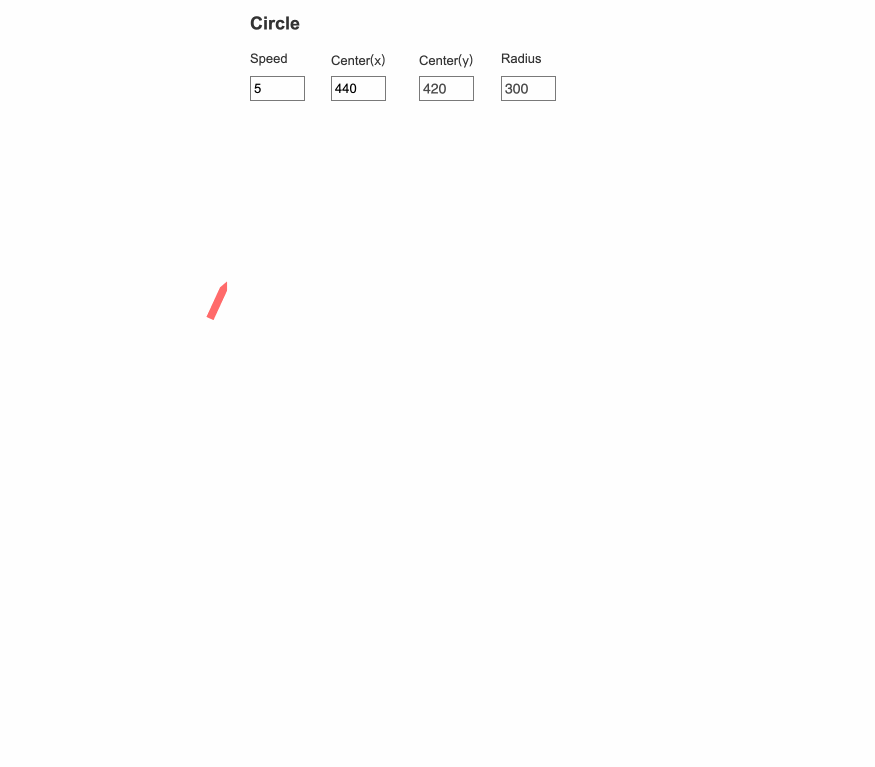1. Make a circular missile trajectory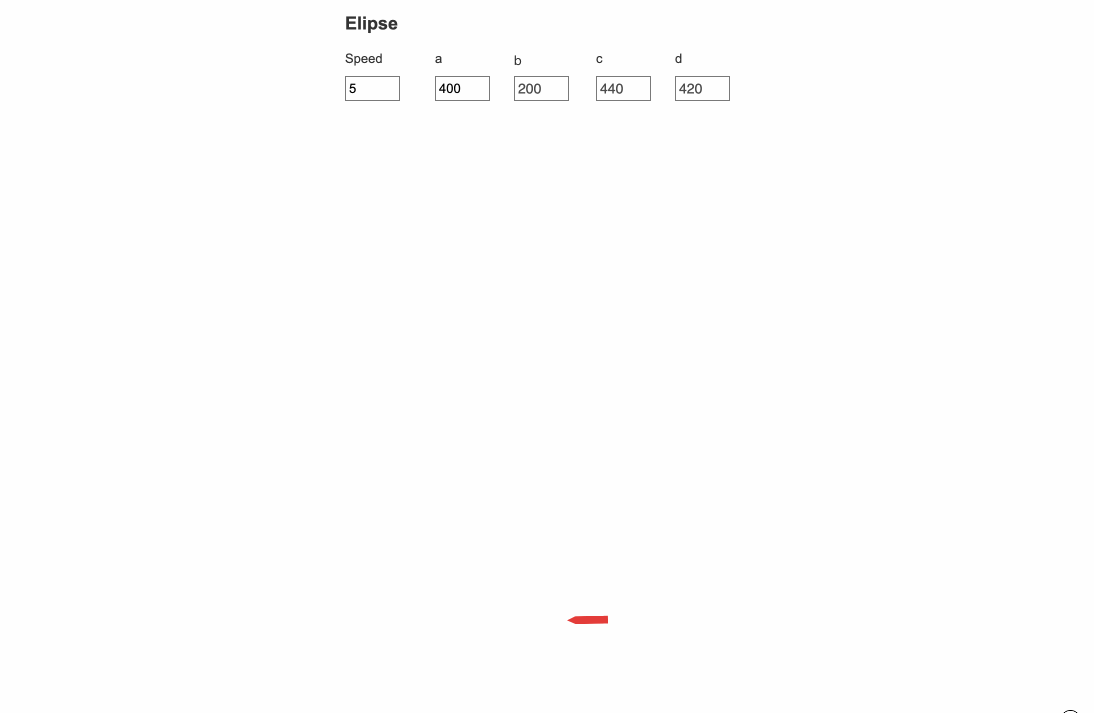2. Making an elliptical missile trajectory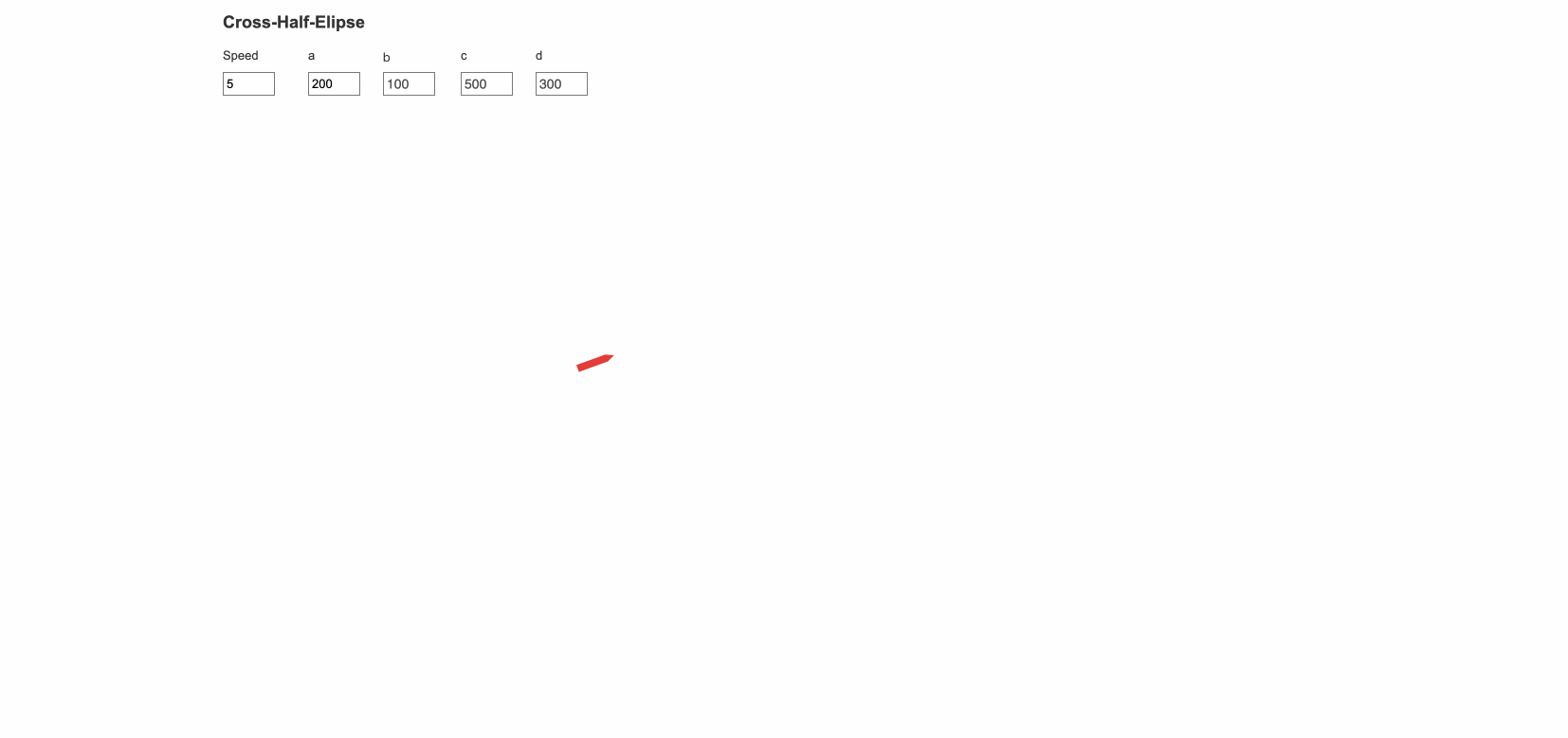3. Make an alternate crossing ellipse track.4. Making a missile collision trajectory

### How to Realize these Features

Calculate the moving distance and rotation angle through circular and elliptical formulas.

### Make a circular missile trajectory

#### Widgets Setup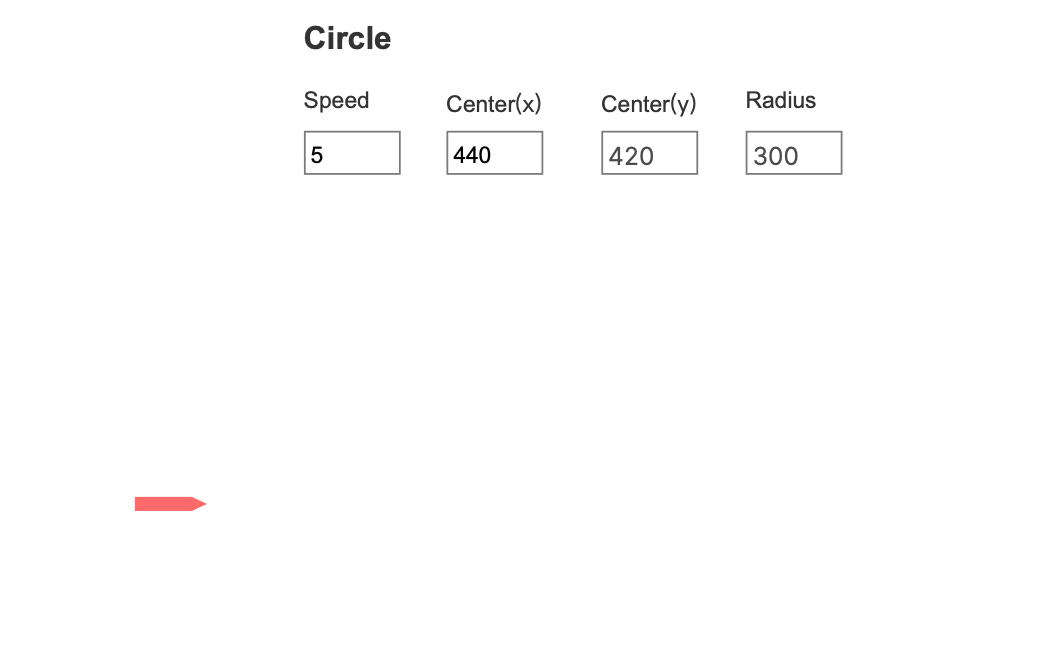Make a missile shape with a combination of rectangles and triangles.  Make labels and text fields for the missile trajectory at the top of the screen.

#### Interactions Setup

For the trajectory of a circle. We just need to know the radius (r) and the position of the center (a, b) of circle, and the speed of the missile . The trajectory equation of a circle is:

(x-a)²+(y-b)²=R²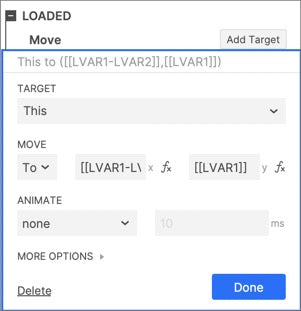First, we move the missile to the left of the circle, that is, a-r, b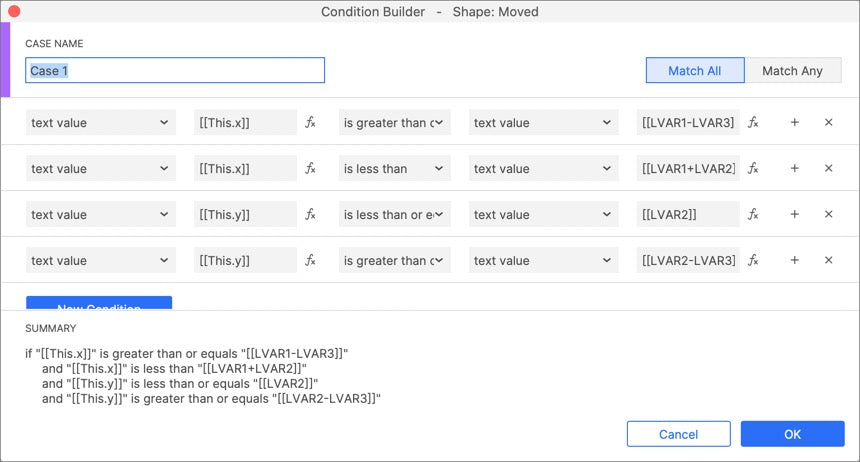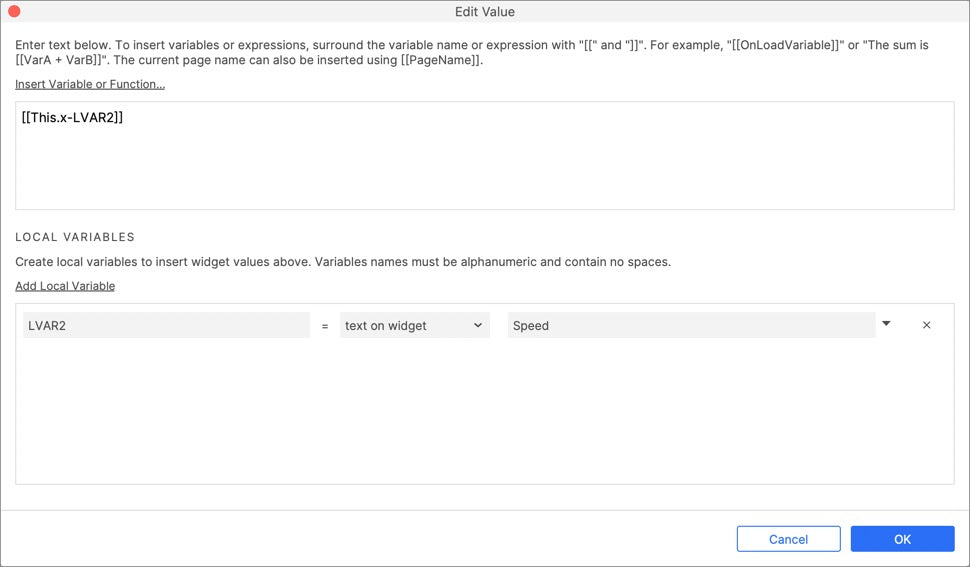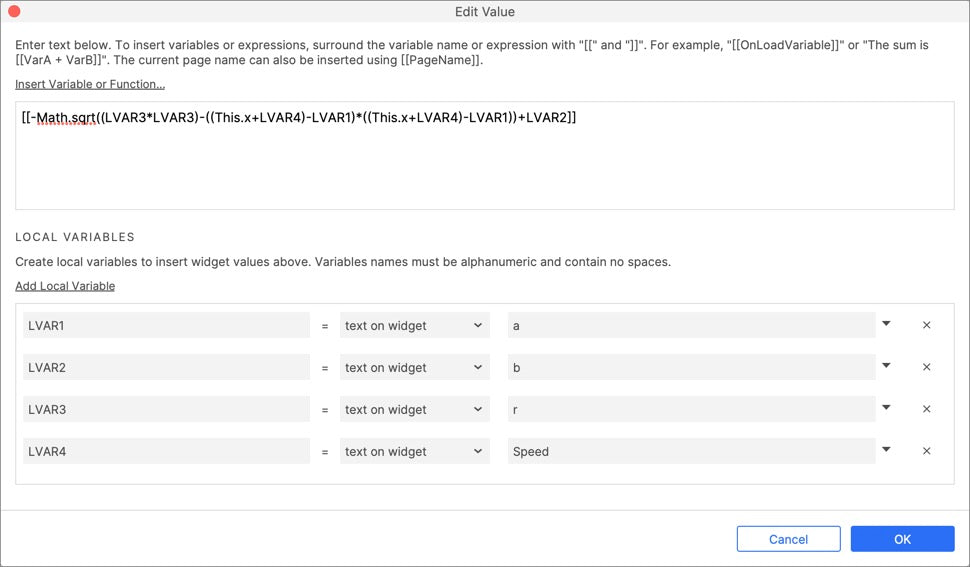Then judge the position of the missile. If it is in the upper half of the circle, the moving distance x = [[This.x-LVAR2]], y= [[Math.sqrt((LVAR3*LVAR3)-((This.x-LVAR4)-LVAR1)*((This.x-LVAR4)-LVAR1))+LVAR2]].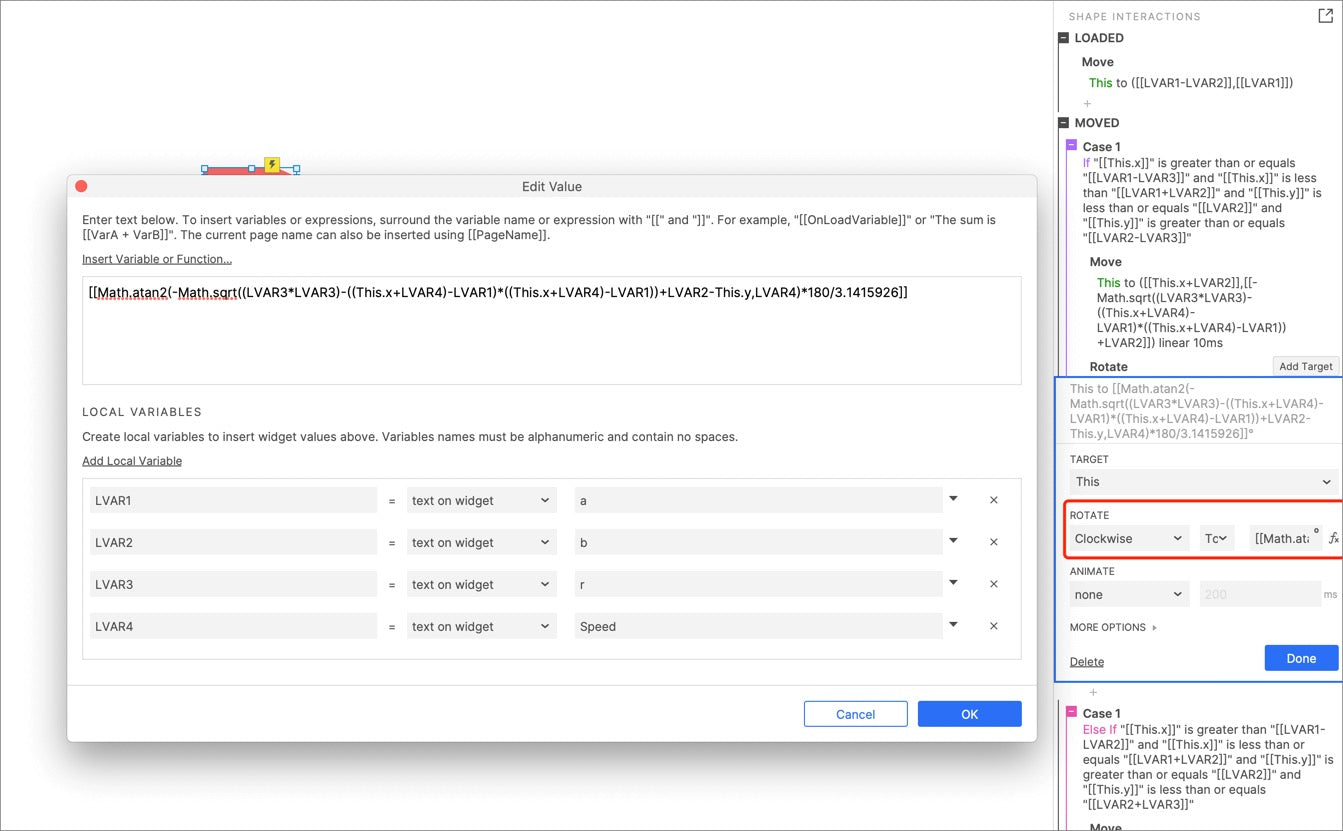The rotation angle is calculated through the formula, and the radian is converted into an angle.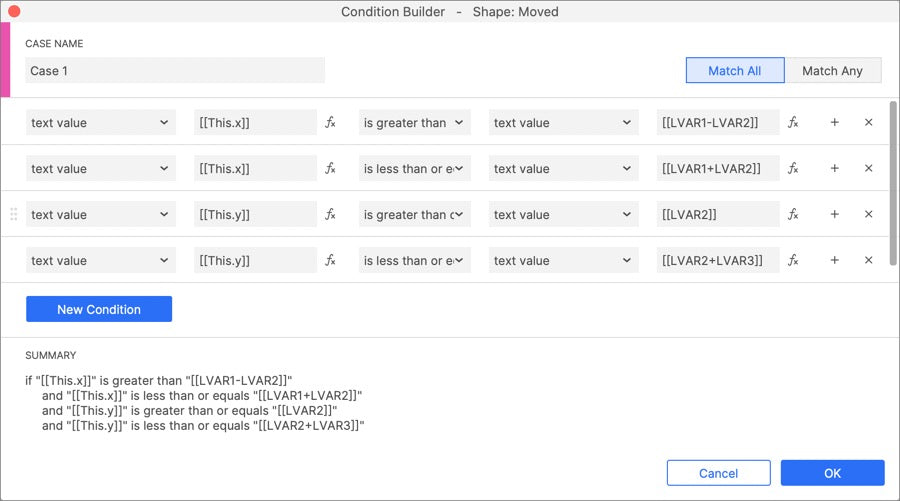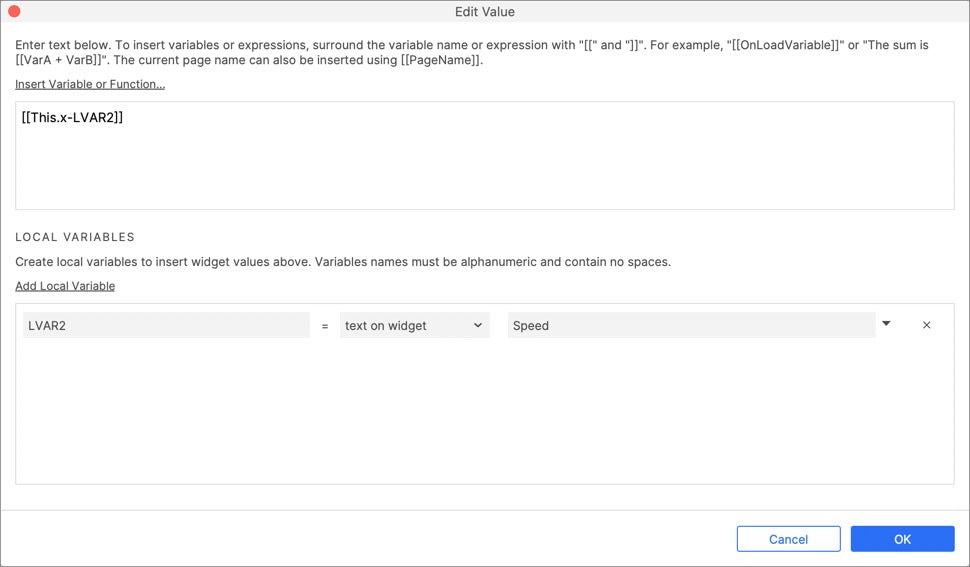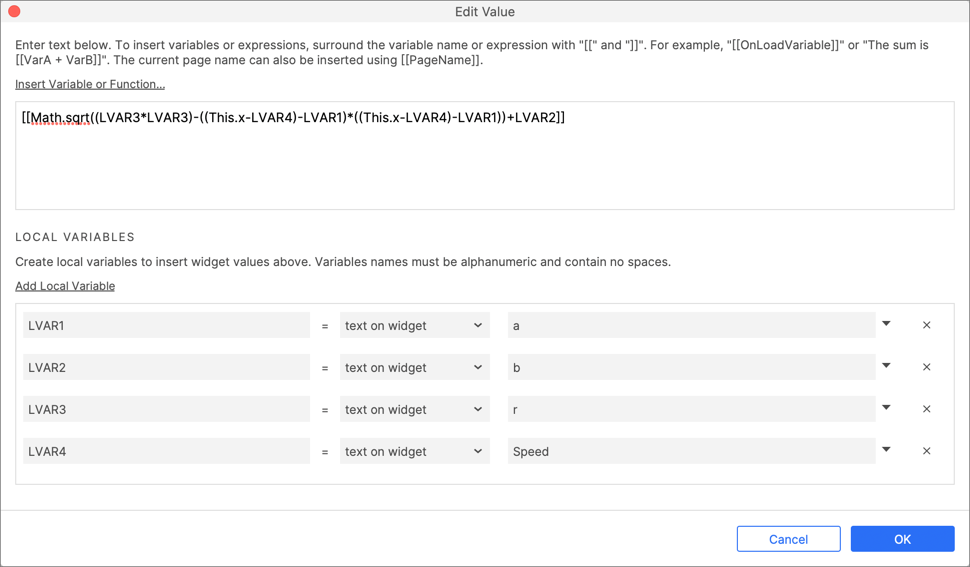If it is in the lower half of the circle, the moving distance x = [[This.x-LVAR2]], y= [[Math.sqrt((LVAR3*LVAR3)-((This.x-LVAR4)-LVAR1)*((This.x-LVAR4)-LVAR1))+LVAR2]].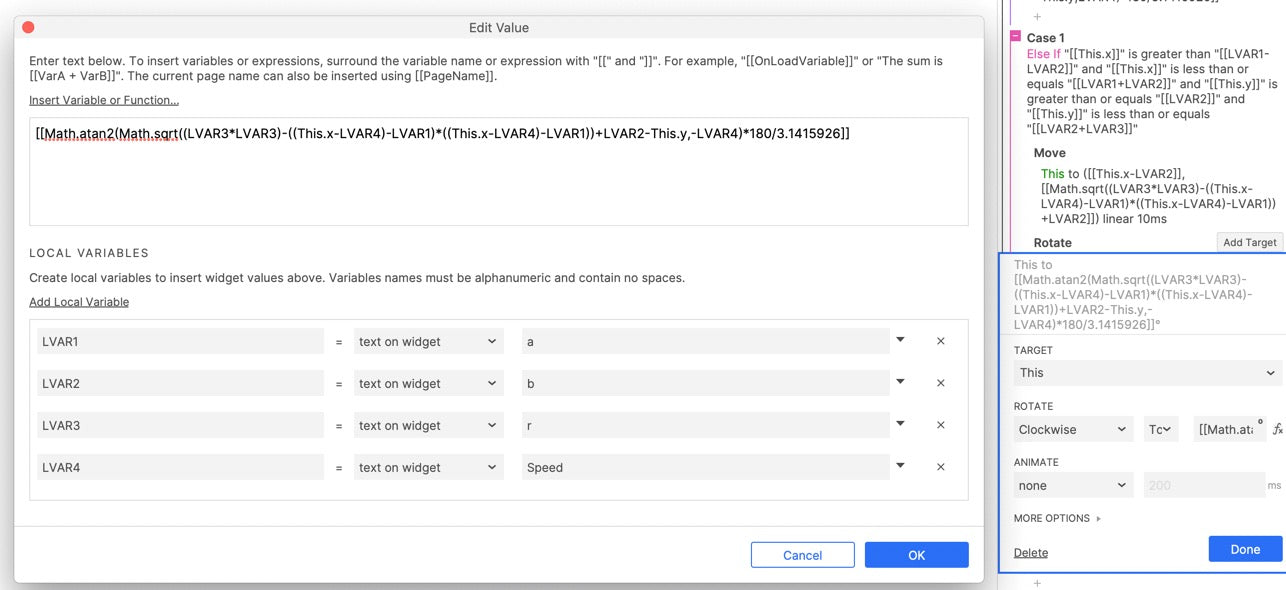The rotation angle is calculated through the formula, and the radian is converted into an angle.

### Make a elliptical missile trajectory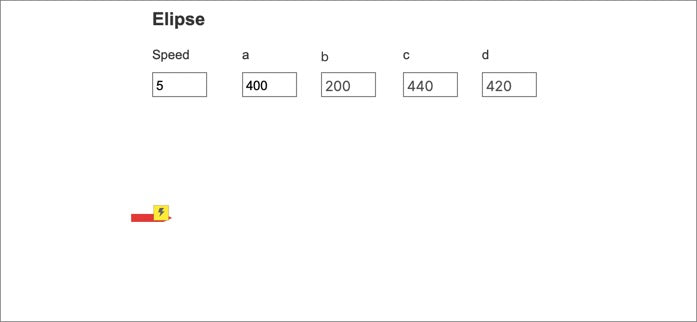The calculation of elliptical trajectory is similar to that of circle, in which half of the length of the long side is a, half of the length of the short side is b, and the position of the center of the circle is c and d.

The calculation formula of trajectory is (x-c) ²/ a ²+ (y-d) ²/ b ²= 1

### Make an alternate crossing ellipse track.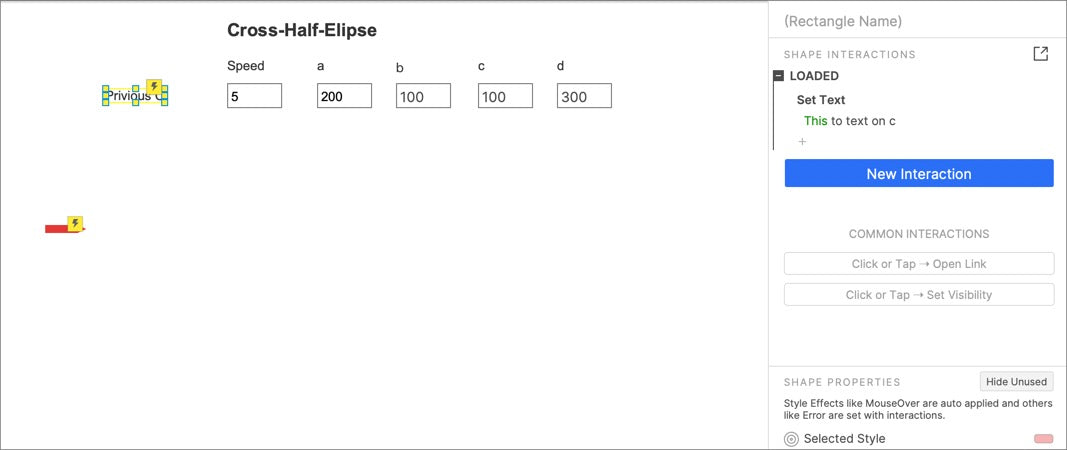The C value of alternate crossing ellipse track will change constantly, so you need to add a parameter: Previous C, which is used to save the previous C value.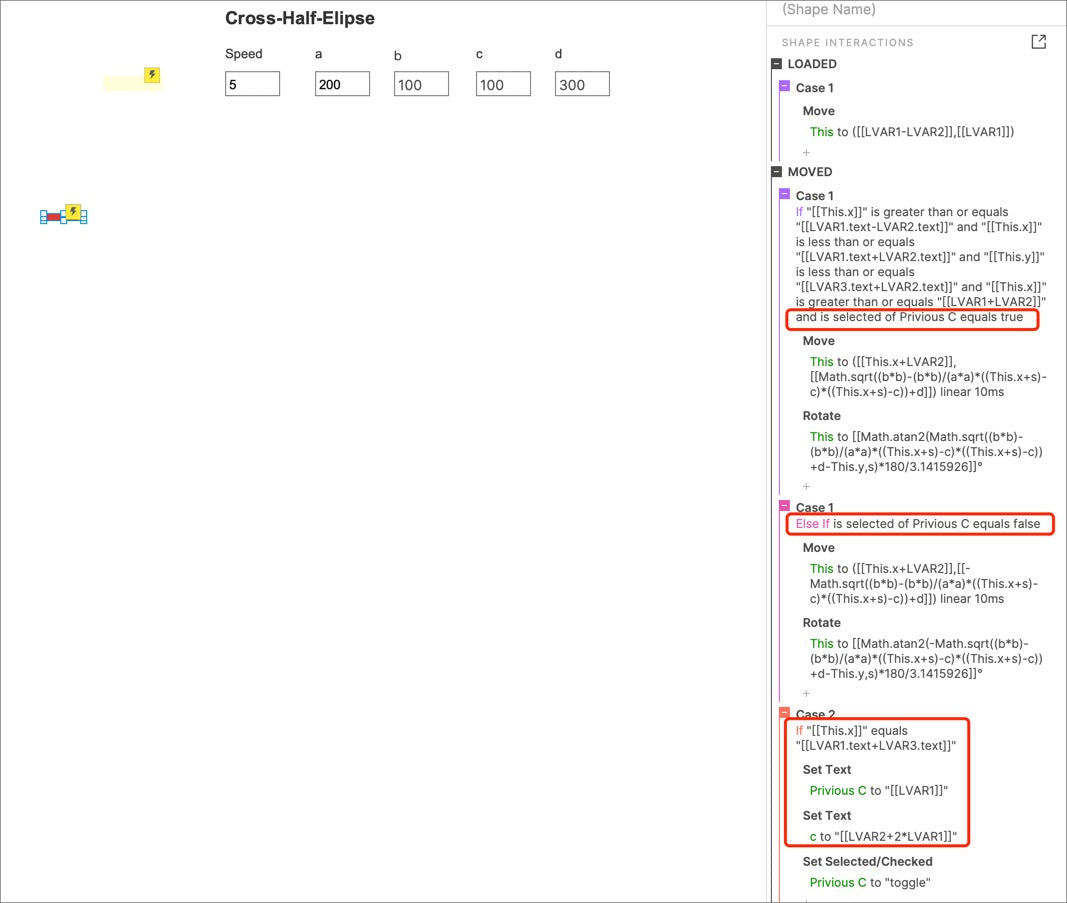The trajectory of the upper and lower ellipses is changed by toggling the selection state of previous C. And the values of C and previous C will change with the value of the missile position.

### Making a missile collision trajectory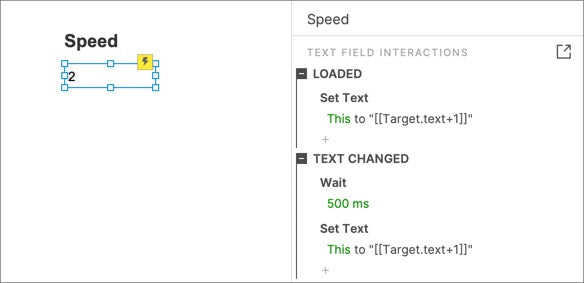The missile collision trajectory needs to add a speed text field, and after a certain time, the speed will increase to simulate the acceleration effect.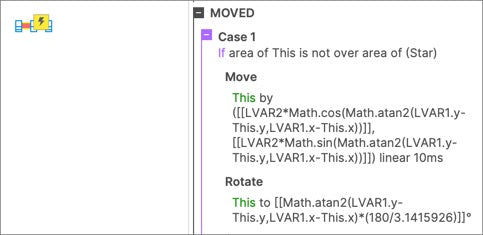The basic principle of missile trajectory is that after a certain time, the coordinates of the missile move to the target and the angle faces the target. Through the trigonometric function, we can calculate them.

If you like the article, please share it with others with page link, thanks for your supporting! ❤

Well Joe @AxureBoutique, a technical writer and teacher, focuses on Axure prototype design and product design.